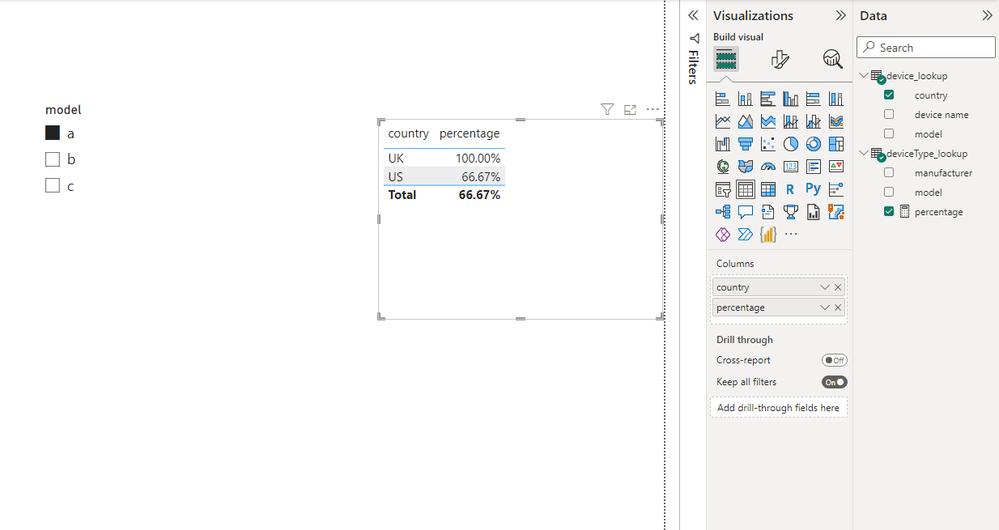cancel
Showing results for
Did you mean:

Fabric is Generally Available. Browse Fabric Presentations. Work towards your Fabric certification with the Cloud Skills Challenge.Frequent Visitor

## How to calculate percentage of data selected by country

Hi there,

I have two tables

device_lookup(device name, model, country)

n1, a, US

n2, a, US

n3, b, US

n4, a, UK

deviceType_lookup(model, manufacturer)

a, lenovo

b, dell

c, acer

with slice to select the mode, how to caculate the percentage of selected devices by country.

ie. if user selected mode = a, i would like to get

US, 66.66%

UK, 100%

1 ACCEPTED SOLUTIONCommunity Support

Hi @elac ,

I create a file for your reference.

The measure I used:

``````percentage = var _count1=CALCULATE(COUNT('device_lookup'[model]),FILTER(ALLSELECTED(device_lookup),[country]=MAX('device_lookup'[country])))
var _count2=CALCULATE(COUNT('device_lookup'[country]),FILTER(ALL(device_lookup),[country]=MAX('device_lookup'[country])))
return DIVIDE(_count1,_count2)``````Best Regards,

Stephen Tao

If this post helps, then please consider Accept it as the solution to help the other members find it more quickly.

4 REPLIES 4Frequent Visitor

Hello  amitchandak,

First, thank you so much for answer.

I tried both measures but it didn't give me the expected result.

device% = divide(countrows(device_lookup), calculate(countrows(device_lookup), removefilters(deviceType_lookup[model]) ) )

device%_2 = divide(countrows(device_lookup), calculate(countrows(device_lookup), all() ) )

However, neither was giving the correct answer.

method 1 always return 100%

method 2 doesn't group on country, it acutally get the percentage on all devices in device lookupAny thoughts? Any help is appreciated!

ElacCommunity Support

Hi @elac ,

I create a file for your reference.

The measure I used:

``````percentage = var _count1=CALCULATE(COUNT('device_lookup'[model]),FILTER(ALLSELECTED(device_lookup),[country]=MAX('device_lookup'[country])))
var _count2=CALCULATE(COUNT('device_lookup'[country]),FILTER(ALL(device_lookup),[country]=MAX('device_lookup'[country])))
return DIVIDE(_count1,_count2)``````Best Regards,

Stephen Tao

If this post helps, then please consider Accept it as the solution to help the other members find it more quickly.Frequent Visitor

Hello Stephen,

This is terrific. It solves my problem perfectly.

If you don't mind, could you tell what is wrong with my original measure?

device%= divide(countrows(device_lookup), calculate(countrows(device_lookup), removefilters(deviceType_lookup[model]) ) )

Thank you so much!

ElacSuper User

@elac , Try a measure like

divide(countrows(Table), calculate(countrows(Table), removefilters(deviceType_lookup[model]) ) )

or

divide(countrows(Table), calculate(countrows(Table), all() ) )Announcements#### Power BI Monthly Update - November 2023

Check out the November 2023 Power BI update to learn about new features.#### Fabric Community News unified experience

Read the latest Fabric Community announcements, including updates on Power BI, Synapse, Data Factory and Data Activator.#### The largest Power BI and Fabric virtual conference

130+ sessions, 130+ speakers, Product managers, MVPs, and experts. All about Power BI and Fabric. Attend online or watch the recordings.Top Solution Authors
Top Kudoed Authors
Users online (3,325)